Hostname: page-component-594f858ff7-hf9kg Total loading time: 0 Render date: 2023-06-10T05:22:44.369Z Has data issue: false Feature Flags: { "corePageComponentGetUserInfoFromSharedSession": true, "coreDisableEcommerce": false, "corePageComponentUseShareaholicInsteadOfAddThis": true, "coreDisableSocialShare": false, "useRatesEcommerce": true } hasContentIssue false

# Instability and transition of a vertical ascension or fall of a free sphere affected by a vertical magnetic field

Published online by Cambridge University Press:  16 November 2018

## Abstract

When the Galileo number is below the first bifurcation, the instability and transition of a vertical ascension or the fall of a free sphere affected by a vertical magnetic field are investigated numerically. A compact model is used to explain that the magnetic field can destabilize the fluid–solid system. When the interaction parameter exceeds a critical value, the sphere trajectory is transitioned from a steady vertical trajectory to a steady oblique one. Furthermore, the trajectory will remain vertical at a sufficiently large magnetic field because of a double effect of the magnetic field on the fluid–solid system. Under the influence of an external vertical magnetic field, four wake patterns at the rear of the sphere are found and the physical behaviour of the free sphere is independent of the density ratio. The wake or trajectory of the free sphere is only determined by the Galileo number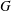$G$ and the interaction parameter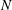$N$. A close relationship between the streamwise vorticity and the sphere motion is found. An interesting ‘agglomeration phenomenon’ is also found, which shows that the vertical velocities are agglomerated into a point for a certain magnetic field regardless of the Galileo number and satisfy a scaling law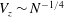$V_{z}\sim N^{-1/4}$, when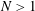$N>1$. The principal results of the present work are summarized in a map of regimes in the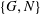$\{G,N\}$ plane.

## JFM classification

Type
JFM Papers
Information
Journal of Fluid Mechanics , 25 January 2019 , pp. 33 - 48

## Access options

Get access to the full version of this content by using one of the access options below. (Log in options will check for institutional or personal access. Content may require purchase if you do not have access.)

## References

Cuevas, S., Smolentsev, S. & Abdou, M. A. 2006 On the flow past a magnetic obstacle. J. Fluid Mech. 553, 227252.CrossRefGoogle Scholar
Davidson, P. A. 2001 An Introduction to Magnetohydrodynamics. Cambridge University Press.CrossRefGoogle Scholar
Dullweber, A., Leimkuhler, B. & McLachlan, R. 1997 Symplectic splitting methods for rigid body molecular dynamics. J. Chem. Phys. 107 (15), 58405851.CrossRefGoogle Scholar
El-Kaddah, N., Patel, A. D. & Natarajan, T. T. 1995 The electromagnetic filtration of molten aluminum using an induced-current separator. JOM J. Miner. Met. Mater. Soc. 47 (5), 4649.CrossRefGoogle Scholar
Ern, P., Risso, F., Fabre, D. & Magnaudet, J. 2012 Wake-induced oscillatory paths of bodies freely rising or falling in fluids. Annu. Rev. Fluid Mech. 44, 97121.CrossRefGoogle Scholar
Fabre, D., Tchoufag, J. & Magnaudet, J. 2012 The steady oblique path of buoyancy-driven disks and spheres. J. Fluid Mech. 707, 2436.CrossRefGoogle Scholar
Giacobello, M., Ooi, A. & Balachandar, S. 2009 Wake structure of a transversely rotating sphere at moderate Reynolds numbers. J. Fluid Mech. 621, 103130.CrossRefGoogle Scholar
Goldsworthy, F. A. 1961 Magnetohydrodynamic flows of a perfectly conducting, viscous fluid. J. Fluid Mech. 11 (4), 519528.CrossRefGoogle Scholar
Horowitz, M. & Williamson, C. H. K. 2010 The effect of Reynolds number on the dynamics and wakes of freely rising and falling spheres. J. Fluid Mech. 651, 251294.CrossRefGoogle Scholar
Hu, H. H., Patankar, N. A. & Zhu, M. Y. 2001 Direct numerical simulations of fluid–solid systems using the arbitrary Lagrangian–Eulerian technique. J. Comput. Phys. 169 (2), 427462.CrossRefGoogle Scholar
Jenny, M., Bouchet, G. & Dušek, J. 2003 Nonvertical ascension or fall of a free sphere in a Newtonian fluid. Phys. Fluids 15 (1), L9L12.CrossRefGoogle Scholar
Jenny, M. & Dušek, J. 2004 Efficient numerical method for the direct numerical simulation of the flow past a single light moving spherical body in transitional regimes. J. Comput. Phys. 194 (1), 215232.CrossRefGoogle Scholar
Jenny, M., Dušek, J. & Bouchet, G. 2004 Instabilities and transition of a sphere falling or ascending freely in a Newtonian fluid. J. Fluid Mech. 508, 201239.CrossRefGoogle Scholar
Johnson, T. A. & Patel, V. C. 1999 Flow past a sphere up to a Reynolds number of 300. J. Fluid Mech. 378, 1970.CrossRefGoogle Scholar
Kanaris, N., Albets, X., Grigoriadis, D. & Kassinos, S. 2013 Three-dimensional numerical simulations of magnetohydrodynamic flow around a confined circular cylinder under low, moderate, and strong magnetic fields. Phys. Fluids 25 (7), 074102.CrossRefGoogle Scholar
Lahjomri, J., Capéran, P. & Alemany, A. 1993 The cylinder wake in a magnetic field aligned with the velocity. J. Fluid Mech. 253, 421448.CrossRefGoogle Scholar
Lighthill, M. J. 1963 Boundary layer theory. In Laminar Boundary Layers (ed. Rosenhead, L.), pp. 46113. Oxford University Press.Google Scholar
Mathai, V., Zhu, X., Sun, C. & Lohse, D. 2017 Mass and moment of inertia govern the transition in the dynamics and wakes of freely rising and falling cylinders. Phys. Rev. Lett. 119 (5), 054501.CrossRefGoogle ScholarPubMed
Mathai, V., Zhu, X., Sun, C. & Lohse, D. 2018 Flutter to tumble transition of buoyant spheres triggered by rotational inertia changes. Nat. Commun. 9 (1), 1792.CrossRefGoogle ScholarPubMed